Anúncio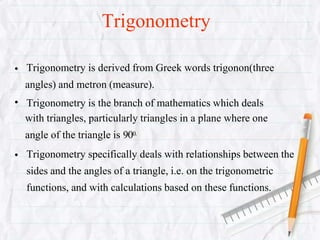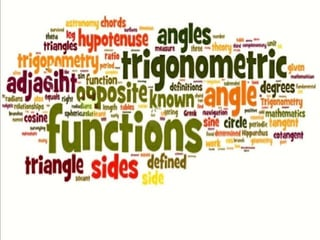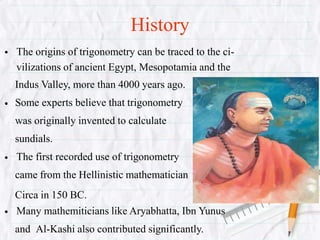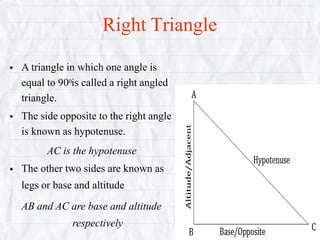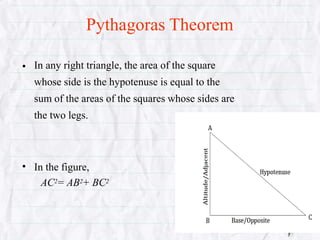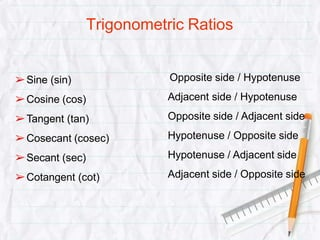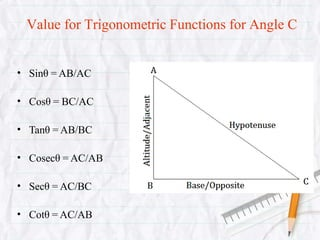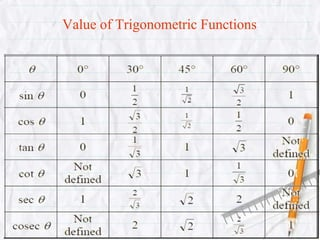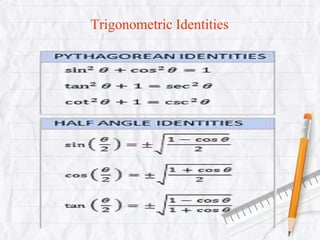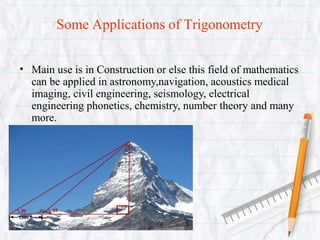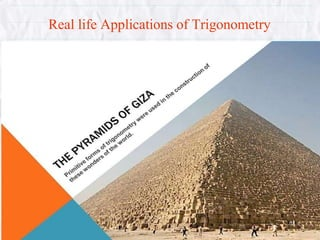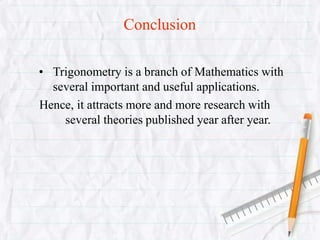1 de 15
Anúncio

### trigonometryabhi-161010073248.pptx

1. • • • Trigonometry is derived from Greek words trigonon(three angles) and metron (measure). Trigonometry is the branch of mathematics which deals with triangles, particularly triangles in a plane where one angle of the triangle is 900. Trigonometry specifically deals with relationships between the sides and the angles of a triangle, i.e. on the trigonometric functions, and with calculations based on these functions. Trigonometry
2. History • • • • The origins of trigonometry can be traced to the ci- vilizations of ancient Egypt, Mesopotamia and the Indus Valley, more than 4000 years ago. Some experts believe that trigonometry was originally invented to calculate sundials. The first recorded use of trigonometry came from the Hellinistic mathematician Circa in 150 BC. Many mathemiticians like Aryabhatta, Ibn Yunus and Al-Kashi also contributed significantly.
3. Right Triangle • • • A triangle in which one angle is equal to 900is called a right angled triangle. The side opposite to the right angle is known as hypotenuse. AC is the hypotenuse The other two sides are known as legs or base and altitude AB and AC are base and altitude respectively
4. Pythagoras Theorem • In any right triangle, the area of the square whose side is the hypotenuse is equal to the sum of the areas of the squares whose sides are the two legs. • In the figure, AC2= AB2+ BC2
5. Trigonometric Ratios ➢Sine (sin) ➢Cosine (cos) ➢Tangent (tan) ➢Cosecant (cosec) ➢Secant (sec) ➢Cotangent (cot) Opposite side / Hypotenuse Adjacent side / Hypotenuse Opposite side / Adjacent side Hypotenuse / Opposite side Hypotenuse / Adjacent side Adjacent side / Opposite side
6. Value for Trigonometric Functions for Angle C • Sinθ = AB/AC • Cosθ = BC/AC • Tanθ = AB/BC • Cosecθ = AC/AB • Secθ = AC/BC • Cotθ = AC/AB
7. Value of Trigonometric Functions
8. Trigonometric Identities
9. Some Applications of Trigonometry • Main use is in Construction or else this field of mathematics can be applied in astronomy,navigation, acoustics medical imaging, civil engineering, seismology, electrical engineering phonetics, chemistry, number theory and many more.
10. Real life Applications of Trigonometry
11. Conclusion • Trigonometry is a branch of Mathematics with several important and useful applications. Hence, it attracts more and more research with several theories published year after year.
12. Thank you!
Anúncio